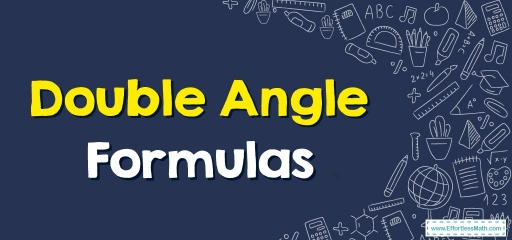# How to Solve Double Angle Identities?

A double angle formula is a trigonometric identity that expresses the trigonometric function $$2θ$$ in terms of trigonometric functions $$θ$$. In this step-by-step guide, you will learn more about double-angle formulas.The double angle formulas are used to find the values of double angles of trigonometric functions using their single angle values. Also, the double-angle formulas can be used to derive the triple-angle formulas.

## A step-by-step guide to double angle formulas

The double angle formulas are the special cases of the sum formulas of trigonometry and some alternative formulas are derived by using the Pythagorean identities. The sum formulas of trigonometry are:

• $$\color{blue}{sin\:\left(A\:+B\right)=sin\:A\:cos\:B\:+\:cos\:A\:sin\:B}$$
• $$\color{blue}{cos\:\left(A\:+\:B\right)=\:cos\:A\:cos\:B\:-\:sin\:A\:sin\:B}$$
• $$\color{blue}{tan\:\left(A\:+\:B\right)=\:\frac{\left(tan\:A\:+\:tan\:B\right)}{\left(1\:-\:tan\:A\:tan\:B\right)}}$$

### What are double-angle formulas?

We derive double-angle formulas of $$sin, cos,$$ and $$tan$$ by substituting $$A=B$$ in each of the above-sum formulas. Also, we will extract some alternative formulas that are derived using Pythagorean identities.

### Double Angle Formulas– Example 1:

If $$tan A= \frac{3}{5}$$, find the values of $$sin\:2A$$.

Solution:

Since the value of $$tan\:A$$ is given, we use the double angle formulas for finding $$sin\:2A$$.

$$sin\:2A=\frac{2\:tan\:A}{1+tan^2A}$$

$$=\frac{2\left(\frac{3}{5}\right)^2}{1+\left(\frac{3}{5}\right)^2}$$

$$=\frac{\frac{18}{25}}{\frac{34}{25}}$$

$$=\frac{18\times 25}{25\times 34}$$

$$=\frac{9}{17}$$

## Exercises forDouble Angle Formulas

1. Find a formula for $$cos(4x)$$ in terms of $$cos x$$.
2. Solve the equation $$sin\:2x\:=\:cos\:x,\:0\:\le \:x\:<\pi$$.
1. $$\color{blue}{8\:cos^4x-8\:cos^2x+1}$$
2. $$\color{blue}{x=\frac{\pi }{2},\frac{\pi }{6},\frac{5\pi }{6}}$$

### What people say about "How to Solve Double Angle Identities? - Effortless Math: We Help Students Learn to LOVE Mathematics"?

No one replied yet.

X
30% OFF

Limited time only!

Save Over 30%

SAVE $5 It was$16.99 now it is \$11.99